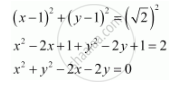CBSE (Arts) Class 11CBSE
Share
Notifications

View all notifications
Books Shortlist
Your shortlist is empty

# Find the Equation of the Circle with Centre (1, 1) and Radius Sqrt2 - CBSE (Arts) Class 11 - Mathematics

Login
Create free account

Forgot password?

#### Question

Find the equation of the circle with centre (1, 1) and radius sqrt2

#### Solution

The equation of a circle with centre (hk) and radius is given as

(x­ – h)2 + (y ­– k)2 = r2

It is given that centre (hk) = (1, 1) and radius (r) =sqrt2

Therefore, the equation of the circle isIs there an error in this question or solution?

#### APPEARS IN

Solution Find the Equation of the Circle with Centre (1, 1) and Radius Sqrt2 Concept: Concept of Circle.
S